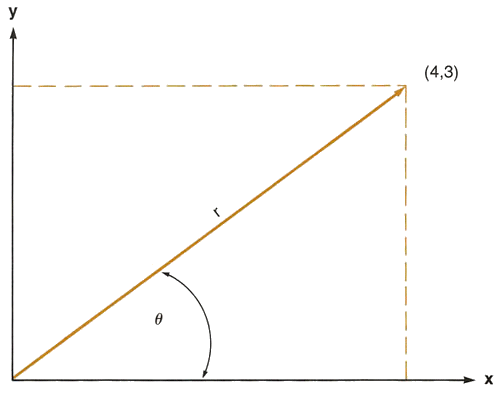Section 6: Functions94
To convert form polar (r, θ) coordinates to rectangular x, y coordinates:
 1. Key in the the value for the angle. 2. Press ENTER  . 3. Key in the the value for the magnitude r. 4. Execute P–R (polar to rectangular). The x-coordinate is placed in the X-register and the y-coordinate is placed in the Y-register. To display the y-coordinate value, press X Y .
When you execute the P–R function, the stack contents are changed...
...from this...           ...to this.

 T t

 T t

 Z z

 Z z

 Y angle θ
P–R
 Y y-coordinate

 X magnitude r

 X x-coordinate

After you execute R–P or P–R , you can press X Y to place the calculated angle θ or the calculated y-coordinate into the X-register for viewing or further calculation.
For example, convert rectangular coordinates (4,3) to polar form with the angle expressed in radians.